Function Repository Resource:

# LoxodromeDistance

Compute the loxodrome distance between two points on a unit sphere

Contributed by: Jan Mangaldan
 ResourceFunction["LoxodromeDistance"][p1,p2] gives the loxodrome distance between points p1 and p2 lying on a sphere centered at the origin.

## Details

The loxodrome, or rhumb line, is a curve of constant bearing/direction on the sphere.
ResourceFunction["LoxodromeDistance"] gives the arclength of the loxodrome connecting p1 and p2.

## Examples

### Basic Examples (1)

The loxodrome distance of two points on a sphere of radius 2:

 In:=Out=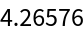### Scope (1)

Loxodrome distance for two randomly chosen points on the unit sphere:

 In:=Out=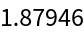### Properties and Relations (5)

Two points on the unit sphere:

 In:=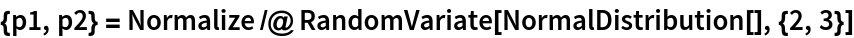Out=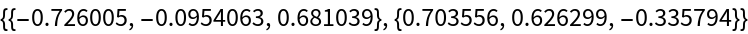Compute the corresponding longitudes and latitudes:

 In:=Out=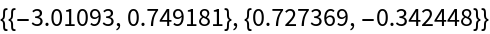Define and visualize the loxodrome joining the two points:

 In:=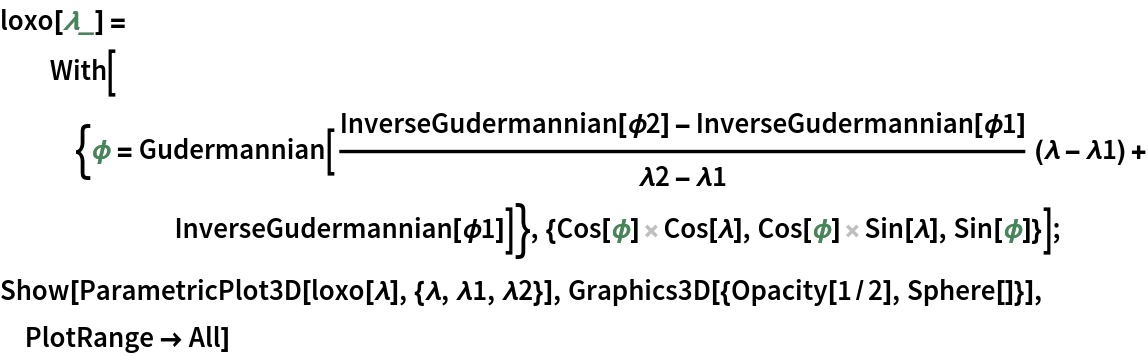Out=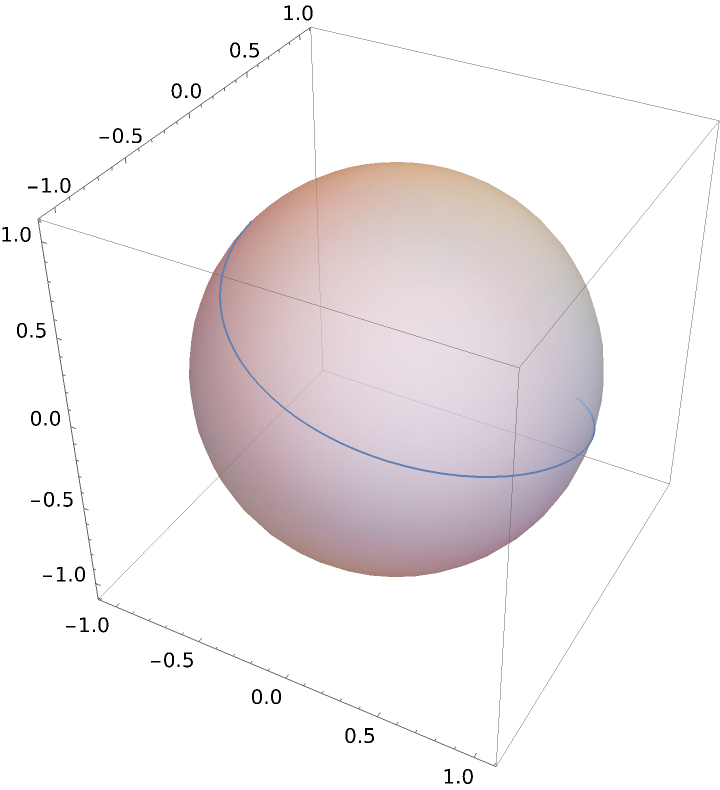Find the arclength of the loxodrome:

 In:=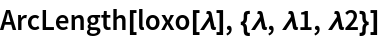Out=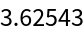This is equivalent to the result of LoxodromeDistance:

 In:=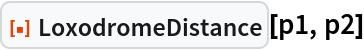Out=## Version History

• 1.0.0 – 19 September 2022